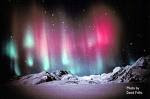## Tuesday, March 9, 2010

### Tutorial: EM 17-2017. Use LHR to determine the force on the charge. The velocity of to positive charge will be the Current direction. a)If charge is stationary, I= 0 , hence no force.
b) Force = 0 , I and B parallel
c) Force into plane
d) Force into plane.
18 Particle B is neutral .
Particle A , Point Force to the centre of the arc (part of a circle). Use LHR , Particle A is positive because the Current direction is the same direction as the velocity of the particle.
Particle C, Point the force to the centre of the arc (part of a circle) . Use LHR , Particle C has current pointing opposite to the velocity of the particle . Hence C is a negative particle.
19. Use LHR, current finger opp to the velocity of electron , the force on the electron is downwards at point of entry. Hence circle down and emerge at RS side.ab) use circular motion formula to find the radius of circle. r = mv/Bq = 4.3 cm .If radius is 7.5 cm , circle will be one quadrant. But r is less than 7.5 cm , so more than one quadrant but less than a semicircle should be drawn.
b) If electric field is applied is such a way that the force is opp to the magnetic force than the electron will experience zero net force and pass through undeflected.
v = E/B, E can be found.
c) Using the equation again , if v is twice than the radius is twice larger. But the direction of force is opposite hence the curve flips up. ( slightly large than one quadrant.
20. As force is perpendicular to B lines, the charged particles circulate round the B lines. It spirals along B lines due to the velocity component parallel to B lines. As charged particles spiral into the Earth near the poles as do B lines ,it collide with the atmospheric molecules producing emission of photons. This colourful display of light is called aurora.